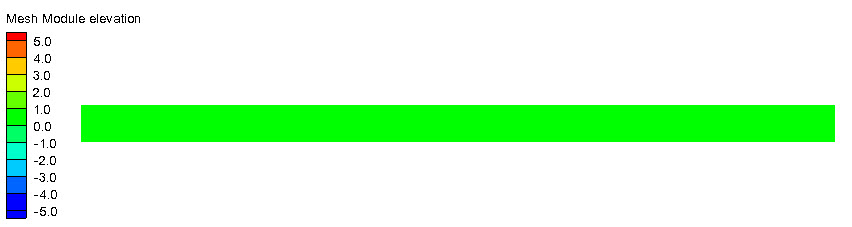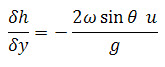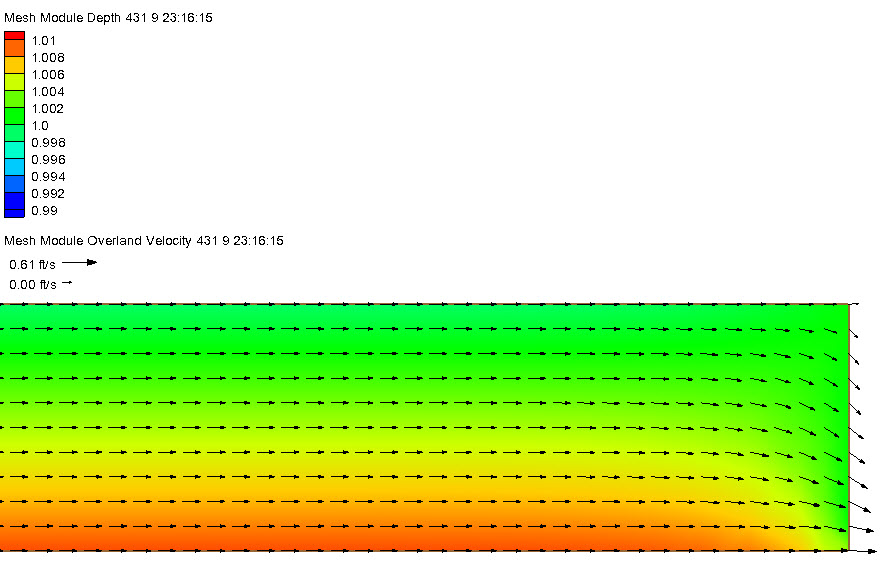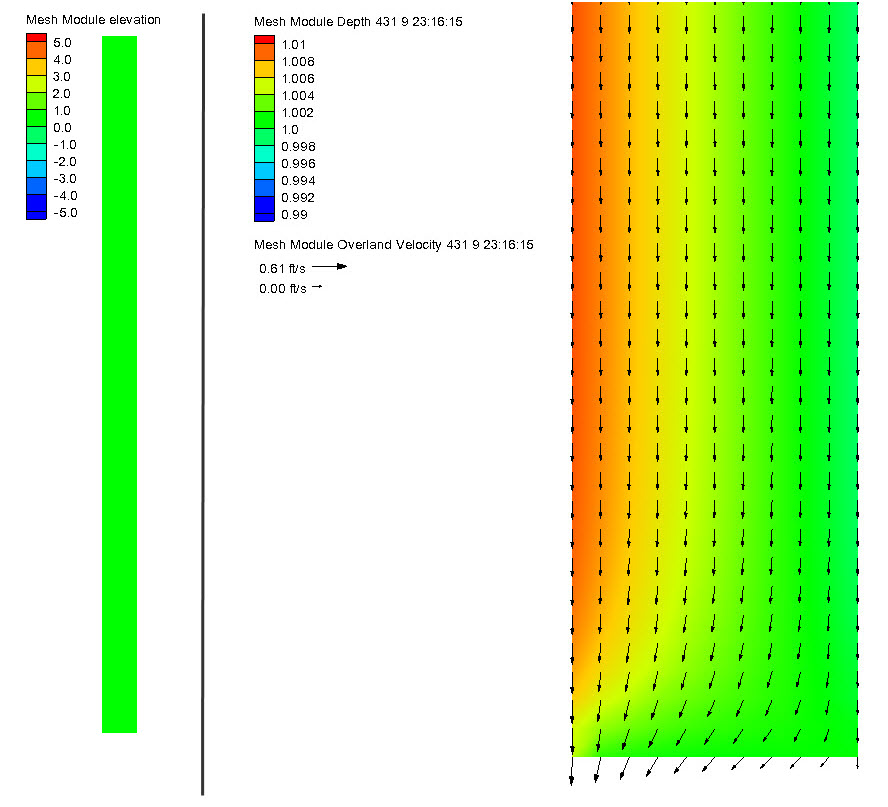## 2D Coriolis

Domain

• rectangular, flat bottomed flume
• 100,000 m length
• 5,000 m width
• 1 m height
• 45 degree latitudeInitial Conditions

• depth of 1 meter
• no flow

Boundary Conditions

• length directed discharge = 1000 cms
• downstream tailwater = 1 m
• Run simulation to steady state

Run Files

• 2D-coriolis_X.3dm
• 2D-coriolis_X.bc
• 2D-coriolis_X.hot
• 2D-coriolis_Y.3dm
• 2D-coriolis_Y.bc
• 2D-coriolis_Y.hot

Results

• Analytic solution for x direction flow
• assume uniform flow
• y velocity = 0.0
• change in u velocity with respect to x = 0.0= -2.0988e-6 m/m

• AdH computed slope = -2.0933e-6
• Velocity shows a curvature to the right which is supported by Coriolis theory• Analytic solution for y direction flow
• assume uniform flow
• x velocity = 0.0
• change in v velocity with respect to y = 0.0
• water surface slope is again = -2.0988e-6 m/m

•
• AdH computes slope = -2.0357e-6
• Velocity shows a curvature to the right which is again supported by Coriolis theory